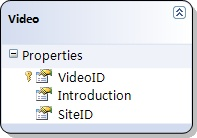## 在Linq to Sql中管理并发更新时的冲突（2）：引发更新冲突

2007-11-20 19:30  Jeffrey Zhao  阅读(3887)  评论(18编辑  收藏1. VideoID：主键，int，自增长
2. Introduction：nvarchar(max)，not null
3. SiteID：int，not null

 VideoID Introduction SiteID 1 Introduction 1 1

LinqToSqlDemoDataContext dataContext = new LinqToSqlDemoDataContext();dataContext.Log = Console.Out;Video video = dataContext.Videos.Single(v => v.VideoID == 1);video.Introduction = "1235";dataContext.SubmitChanges(); Console.ReadLine();

SELECT [t0].[VideoID], [t0].[Introduction], [t0].[SiteID]FROM [dbo].[Video] AS [t0]WHERE [t0].[VideoID] = @p0-- @p0: Input Int (Size = 0; Prec = 0; Scale = 0) -- Context: SqlProvider(Sql2005) Model: AttributedMetaModel Build: 3.5.21004.1UPDATE [dbo].[Video]SET [Introduction] = @p3WHERE ([VideoID] = @p0) AND ([Introduction] = @p1) AND ([SiteID] = @p2)-- @p0: Input Int (Size = 0; Prec = 0; Scale = 0) -- @p1: Input NVarChar (Size = 14; Prec = 0; Scale = 0) [Introduction 1]-- @p2: Input Int (Size = 0; Prec = 0; Scale = 0) -- @p3: Input NVarChar (Size = 16; Prec = 0; Scale = 0) [New Introduction]-- Context: SqlProvider(Sql2005) Model: AttributedMetaModel Build: 3.5.21004.1

try{    LinqToSqlDemoDataContext dataContext = new LinqToSqlDemoDataContext();    Video video = dataContext.Videos.Single(v => v.VideoID == 1);    // 在下一行代码上设断点，并在运行时改变数据库内纪录的值。    video.Introduction = "12356";    dataContext.SubmitChanges();}catch (ChangeConflictException e){    Console.WriteLine(e.Message);}Consoleonsole.ReadLine();

Row not found or changed.

LinqToSqlDemoDataContext dataContext = new LinqToSqlDemoDataContext();dataContext.Log = Console.Out;Video video = new Video();video.VideoID = 1;dataContext.Videos.Attach(video, false);video.Introduction = "123";dataContext.SubmitChanges();

UPDATE [dbo].[Video]SET [Introduction] = @p0WHERE 0 = 1-- @p0: Input NVarChar (Size = 3; Prec = 0; Scale = 0) -- Context: SqlProvider(Sql2005) Model: AttributedMetaModel Build: 3.5.21004.1